Question

The complex [Fe(H2O)6]3+ has a maximum absorbance at 724 nm.

a.Calculate the crystal field splitting energy, in kJ, for the complex.

b.Predict the colour of the complex.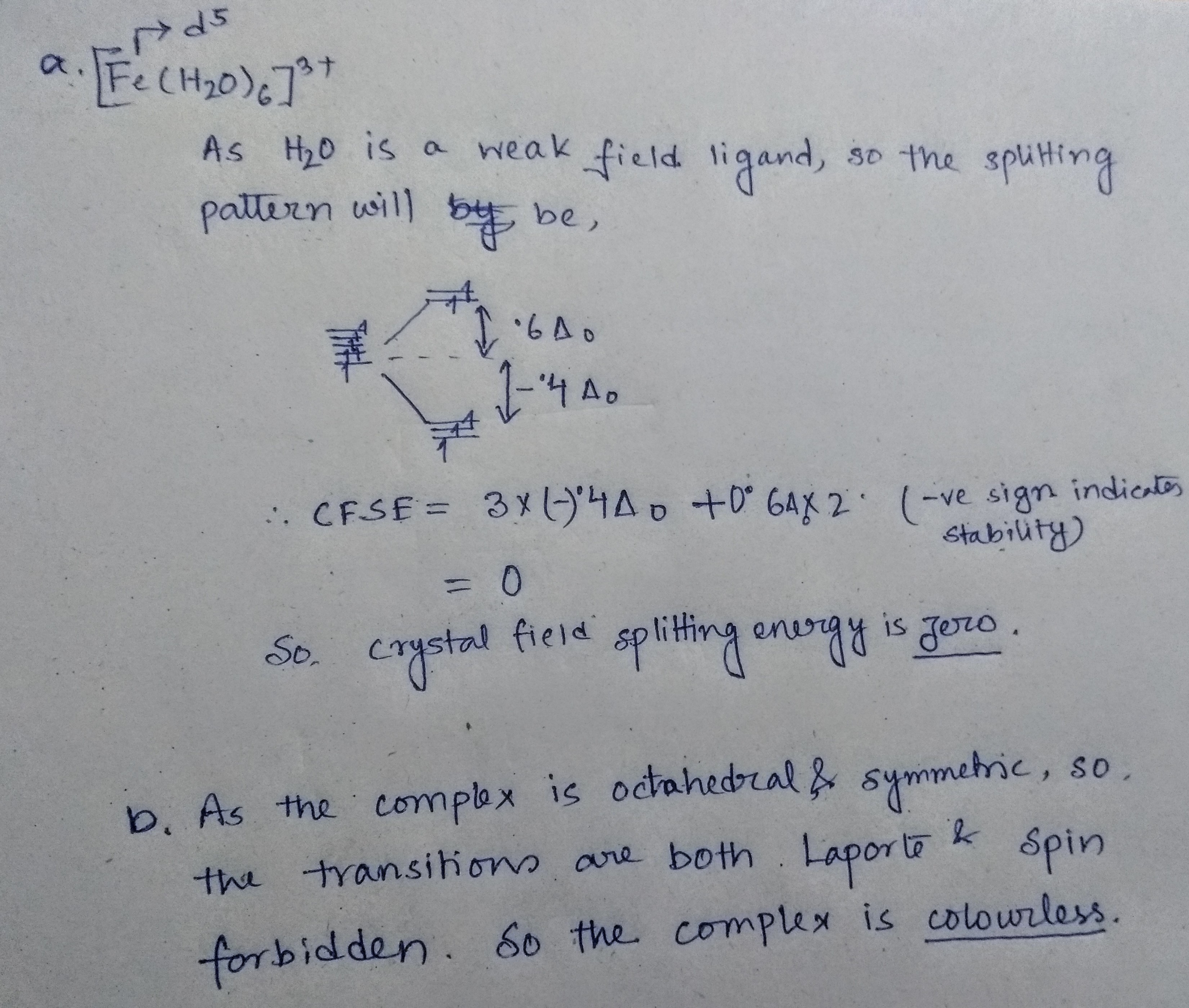please rate it, if you understand the answer

#### Earn Coins

Coins can be redeemed for fabulous gifts.

Similar Homework Help Questions
• ### A solution containing [Fe(CN)6]3" has its maximum absorbance at 305 nm. Calculate the crystal field splitting...

A solution containing [Fe(CN)6]3" has its maximum absorbance at 305 nm. Calculate the crystal field splitting energy for the compound and predict its colour

• ### CE IZOLIUM The (RhC16]has its maximum absorbance at 495 nm. 1. Calculate the crystal field splitting...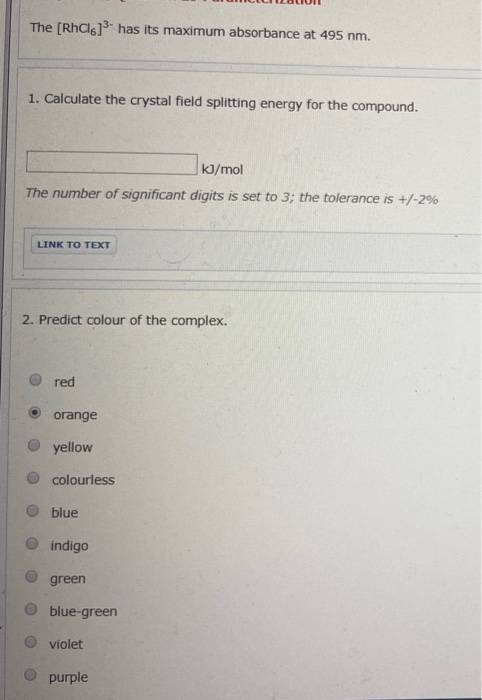CE IZOLIUM The (RhC16]has its maximum absorbance at 495 nm. 1. Calculate the crystal field splitting energy for the compound. kJ/mol The number of significant digits is set to 3; the tolerance is +/-2% LINK TO TEXT 2. Predict colour of the complex. red orange yellow O colourless O blue indigo O green blue-green violet O purple

• ### A ?1 octahedral complex is found to absorb visible light, with the absorption maximum occurring at 499 nm . Calculate the crystal-field splitting energy, Δ , in kJ/mol.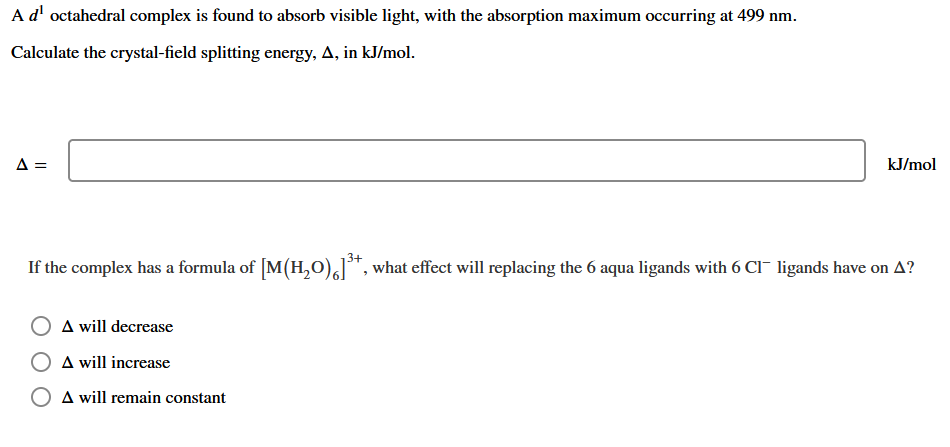A ?1 octahedral complex is found to absorb visible light, with the absorption maximum occurring at 499 nmCalculate the crystal-field splitting energy, Δin kJ/mol.

• ### Calculate the crystal field stabilization energy valiue for the complex Cr(NH)J*. The crystal field splitting energy...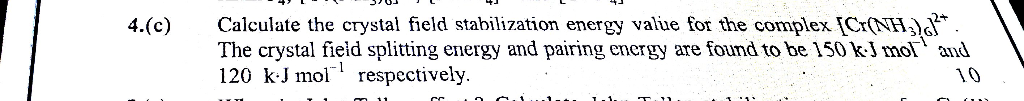Calculate the crystal field stabilization energy valiue for the complex Cr(NH)J*. The crystal field splitting energy and pairing energy are found to be 150 kJ mol and 120 kJ mol respectively. 4.(c) 10 cc. Calculate the crystal field stabilization energy valiue for the complex Cr(NH)J*. The crystal field splitting energy and pairing energy are found to be 150 kJ mol and 120 kJ mol respectively. 4.(c) 10 cc.

• ### The extent of crystal field splitting is often determined from spectra. Given the wavelength (7.) of...The extent of crystal field splitting is often determined from spectra. Given the wavelength (7.) of maximum absorption, 2. = 295 nm, find the crystal field splitting energy (A), in kJ/mol, for the complex ion (Rh(NH3).13+. kJ/mol

• ### The [CrCl_6]^3- ion has a maximum in its absorption spectrum at 735 nm. Calculate the crystal...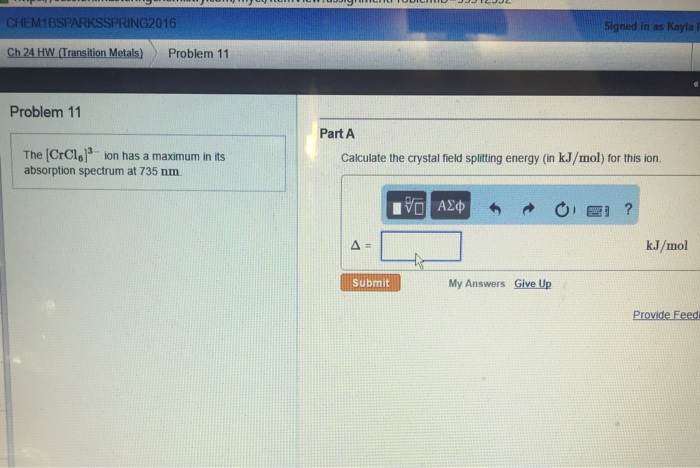The [CrCl_6]^3- ion has a maximum in its absorption spectrum at 735 nm. Calculate the crystal field splitting energy (in kJ/mol) for this ion.

• ### 13. An octahedral transition metal complex with a d' electron configuration absorbs light of wavelength equal to 650 nm. a. what is the magnitude of the octahedral crystal field splitting par...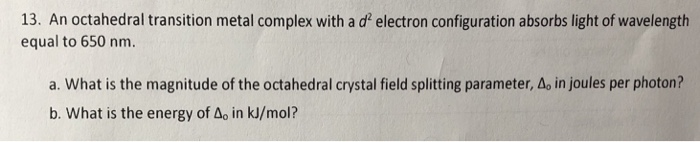13. An octahedral transition metal complex with a d' electron configuration absorbs light of wavelength equal to 650 nm. a. what is the magnitude of the octahedral crystal field splitting parameter, Δο in joules per photon? b. What is the energy of Ao in kJ/mol? 13. An octahedral transition metal complex with a d' electron configuration absorbs light of wavelength equal to 650 nm. a. what is the magnitude of the octahedral crystal field splitting parameter, Δο in joules per...

• ### .. Fe is identified by the rapid formation of the intensely colored pentaaquathio-cyanatoiron (III) complex ion,...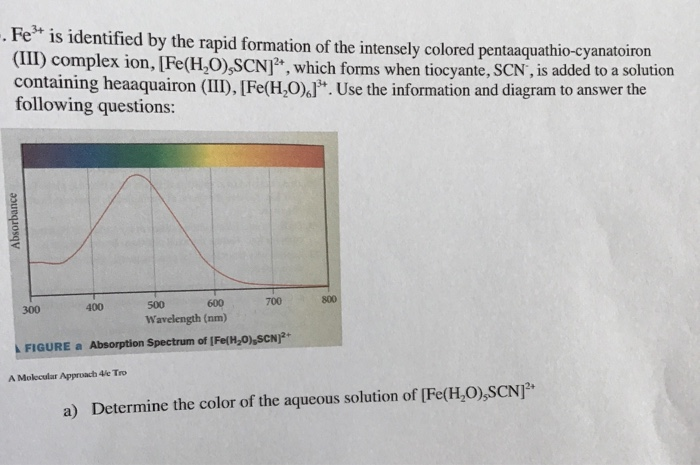.. Fe is identified by the rapid formation of the intensely colored pentaaquathio-cyanatoiron (III) complex ion, [Fe(H,O),SCN , which forms when tiocyante, SCN, is added to a solution containing heaaquairon (III), [Fe(H,0).J. Use the information and diagram to answer the following questions: Absorbance 300 400 500 600 700 Wavelength (nm) FIGURE a Absorption Spectrum of [Fe(H20),SCN)2+ A Molecular Approach 4le Tro a) Determine the color of the aqueous solution of [Fe(HO),SCN b) Calculate the crystal field splitting energy of [Fe(H,0),SCN]...

• ### An octahedral metal complex absorbs light with wavelength 580 nm. a. What is the crystal field...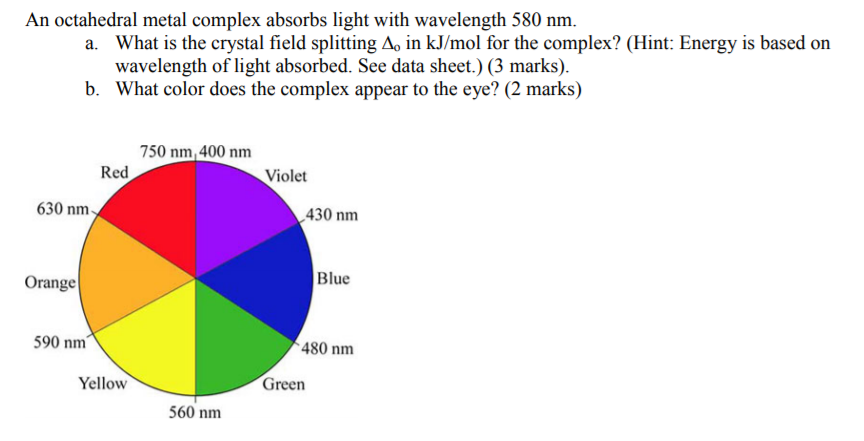An octahedral metal complex absorbs light with wavelength 580 nm. a. What is the crystal field splitting A, in kJ/mol for the complex? (Hint: Energy is based on wavelength of light absorbed. See data sheet.) (3 marks) b. What color does the complex appear to the eye? (2 marks) 750 nm, 400 nm Red Violet 630 nm 430 nm Blue Orange 590 nm 480 nm Yellow Green 560 nm

• ### Which of the following complexes has the largest crystal field splitting of the d orbitals? A....

Which of the following complexes has the largest crystal field splitting of the d orbitals? A. [Fe(H2O)6]3+ B. [FeF6]3– C. [Fe(NH3)6]3+ D. [FeI6]3– E. [Fe(CO)6]3+